# Customer Lifetime Value Calculation

When considering the customer lifetime value, the most curious things that come to mind are what is the customer lifetime value definition and how about the formula to calculate it?

I have explained in detail the definition and required formulas to calculate customer lifetime value in my previous medium article.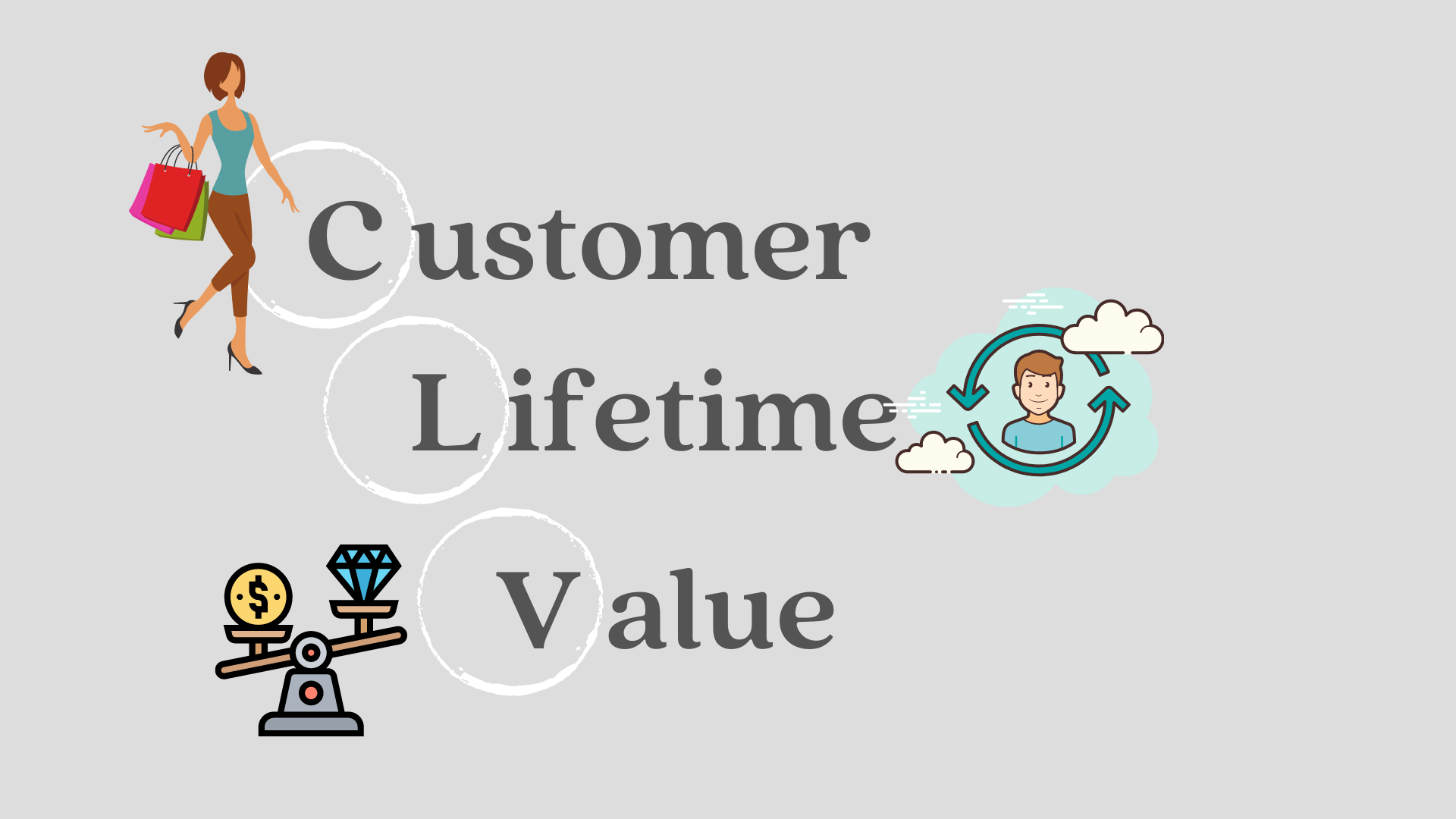Customer Lifetime Value(CLTV)

Throughout this article, customer lifetime value example for the calculation will be discussed and customers will be divided into 4 segments according to CLTV value.

Data Set Information:

This Online Retail II data set contains all the transactions occurring for a UK-based and registered, non-store online retail between 01/12/2009 and 09/12/2011. The company mainly sells unique all-occasion gift-ware. Many customers of the company are wholesalers.

Invoice: Invoice number. Nominal. A 6-digit integral number uniquely assigned to each transaction. If this code starts with the letter ‘c’, it indicates a cancellation.

StockCode: Product (item) code. Nominal. A 5-digit integral number uniquely assigned to each distinct product.

Description: Product (item) name.

Quantity: The quantities of each product (item) per transaction.

InvoiceDate: Invice date and time. Numeric. The day and time when a transaction was generated.

UnitPrice: Unit price. Numeric. Product price per unit in sterling.

CustomerID: Customer number. Nominal. A 5-digit integral number uniquely assigned to each customer.

Country: Country name. Nominal. The name of the country where a customer resides.

Required dataset was read and the necessary library was imported. Note: Libraries will be imported where they necessary during the calculation.

DATA PREPARATION

Calculation started with data preparation. First of all, since the letter “C” indicates the cancelation the values which do not contains “C” in the invoice code are selected.

Quantity value is greater than zero was confirmed and missing values ​​were dropped.

Total price variable which is required for customer lifetime value calculation is created by using Quantity and Price values. Then, new dataframe is created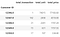cltv_df.head()

At the end of data preparation step dataset is ready for CLTV calculation.

Let’s remember required formulas for CLTV calculation: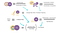Summary of CLV Calculation

Average order value calculation:

Purchase frequency calculation:

Repeat rate: If total transaction is bigger than one means that these customers were purchased more than once.

Churn rate: Also known as the customer churn, is the rate at whivh customers stop doing business with an institution.

Repeat rate & churn rate calculation:

Profit Margin: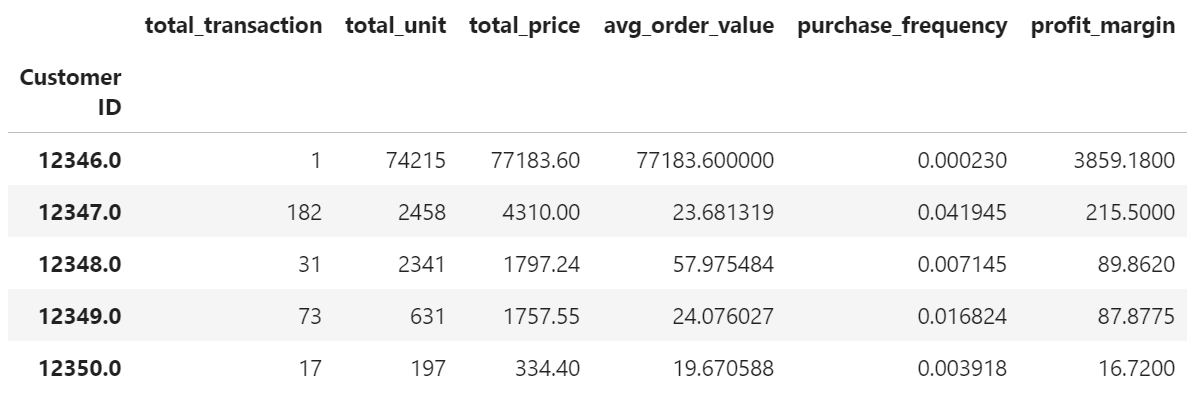cltv_df.head()

Customer value (CV) is calculated by using average order value and purchase frequency. Then by using CV, profit margin and churn rate CLTV is calculated.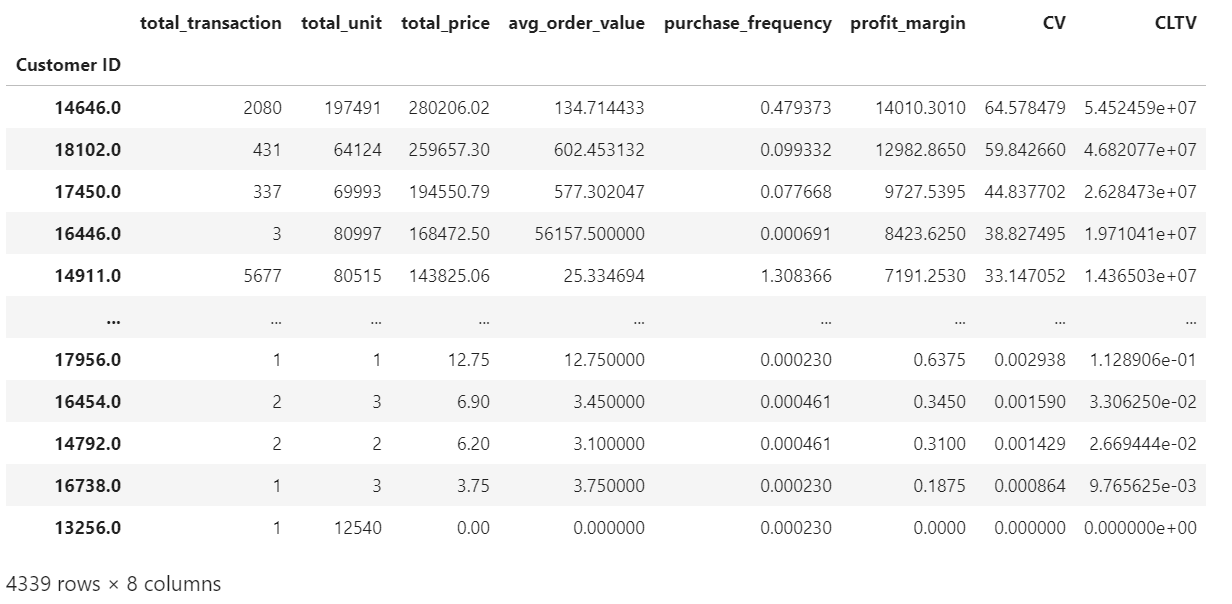cltv_df.sort_values(“CLTV”, ascending = False)

CLTV values are converted between 1–100 scale to better observe the results.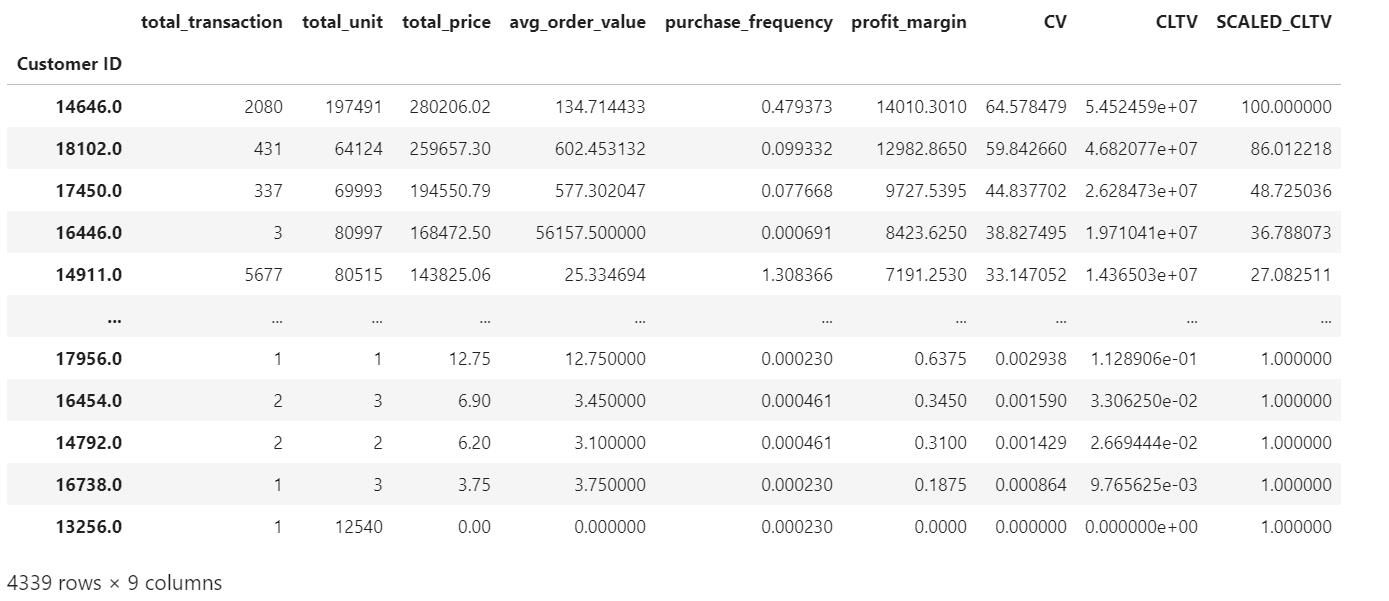cltv_df.sort_values(“CLTV”, ascending=False)

For comparison of values, variables are chosen from dataframe and values are sorted by “SCALED_CLTV”.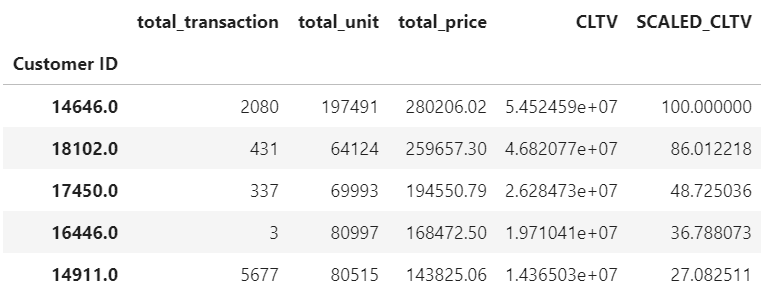sort_values(by = “SCALED_CLTV”,ascending= False).head()

Finally, by using SCALED_CLTV values, segments are created with qcut. These created segments are helpful to make the customer experience personalized.

Total transaction, total unit, total price, CLTV and SCALED CLTV values are interpreted with their count, sum, and mean values.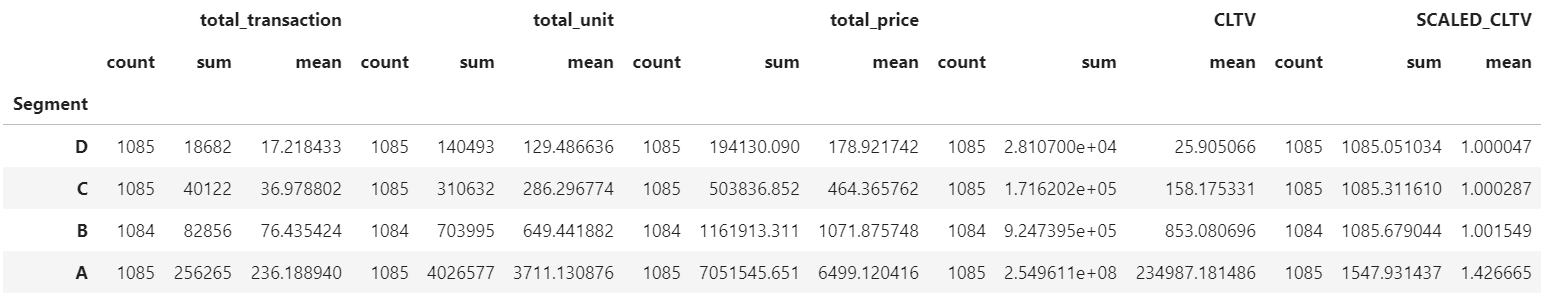cltv_df.groupby(“Segment”)[[“total_transaction”, “total_unit”, “total_price”, “CLTV”, “SCALED_CLTV”]].agg( {“count”, “mean”, “sum”})

## More from İkbal Arslan

Life is a learning process https://www.linkedin.com/in/ikbal-arslan/